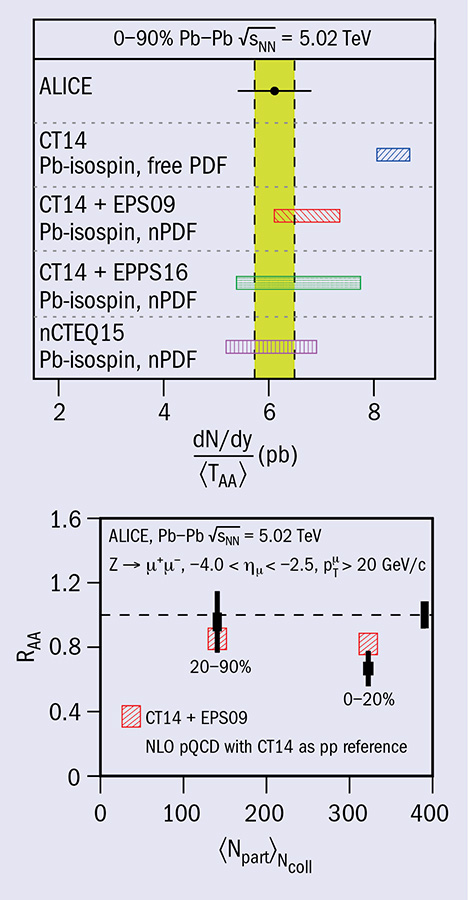# ALICE probes partons inside lead nuclei

1 June 2018Top: invariant yield of dimuons from Z-boson production in the rapidity range 2.5 < y < 4.0 divided by the average nuclear overlap function in the 0–90% centrality class in PbPb collisions at 5.02 TeV. The horizontal bar represents the statistical uncertainty, while the yellow band represents the systematic uncertainty, and the result is compared to theoretical calculations with and without nuclear modification of the PDFs. Bottom: nuclear modification factor as a function of the collision centrality: vertical error bars are statistical, while boxes represent systematic uncertainties.

The large centre-of-mass energy and luminosity of the LHC have made possible the first measurements of electroweak-boson production in ultrarelativistic heavy-ion collisions. The production cross sections for such processes in proton–proton (pp) collisions are known with high precision, and indeed have been suggested as “standard candles” for luminosity measurements at the LHC. Since the electroweak bosons and their leptonic decay products do not interact strongly with the hot and dense quark–gluon matter produced in heavy-ion collisions, they can also be used as a reference in this environment. Here, the production rates of W and Z bosons directly probe initial-state effects, such as the u- and d-quark density (isospin) and the difference between the parton density functions (PDFs) of nucleons that are bound in nuclei and those that are free. These effects are studied by comparing the measurements in lead–lead (PbPb) collisions to the results from pp collisions, taking their different collision-impact parameters into account.

The ALICE experiment has measured, for the first time, Z-boson production at large rapidity in PbPb collisions at a centre-of-mass energy of 5.02 TeV per nucleon pair (figure, top). The measurement was compared with theoretical calculations at next-to-leading order, considering a combination of proton and neutron PDFs to account for the isospin of the lead nucleus. Those calculations that include a nuclear modification of the PDFs (through three different parameterisations) describe the data well, while the calculation using free proton and neutron PDFs overestimates the data by 2.3 standard deviations.

The Z production rate was studied as a function of rapidity and collision centrality. To study the radial dependence of the nuclear effects, the data sample was divided into two different centrality classes and the nuclear modification factor RAA was evaluated by dividing the normalised yields by the theoretical pp cross-section reference (figure, bottom). The measurements are well described by the calculations using an impact-parameter-dependent nuclear PDF, and the data point in the 0–20% most central collisions deviates from the predictions with free PDFs by three standard deviations.

The Z-boson measurements at large rapidity in PbPb collisions at LHC energies are well-described by calculations that include nuclear modifications of the PDFs. These have been inferred mostly from deep-inelastic scattering experiments at lower energies, while the predictions using free PDFs deviate from data. The data from the upcoming PbPb data-taking period in November will allow ALICE to improve the precision of the electroweak-boson measurements and provide more precise information on the modification of PDFs in nuclei.